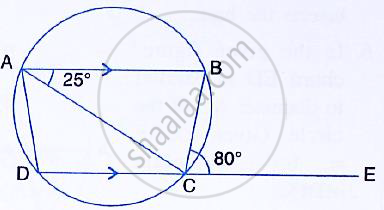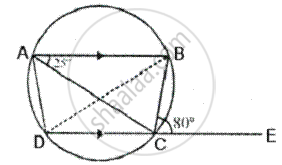Share

In the Given Figure, Ab is Parallel to Dc ∠Bce = 80° and ∠Bac = 25°.Find: (I) ∠Cad (Ii) ∠Cbd (Iii) ∠Adc - ICSE Class 10 - Mathematics

Question

In the given figure, AB is parallel to DC ∠BCE = 80° and ∠BAC = 25°.Find:

SolutionIn the given figure,
ABCD is a cyclic quad in which AB ∥ DC
∴ ABCD is an isosceles trapezium
∴ AD = BC

Ext. ∠BCE = ∠BAD  [Exterior angle of a cyclic qud is equal to interior opposite angle]

∴ ∠BAD = 80°      [ ∵ ∠BCE = 80°]

But ∠BAC = 25°

= 80° - 25°
= 55°

(ii) ∠CBD = ∠CAD   [Angle of the same segment]
= 55°

(iii) ∠ADC = ∠BCD   [Angles of the isosceles trapezium]

= 180° - ∠BCE
= 180° - 80°
= 100°

Is there an error in this question or solution?
Solution In the Given Figure, Ab is Parallel to Dc ∠Bce = 80° and ∠Bac = 25°.Find: (I) ∠Cad (Ii) ∠Cbd (Iii) ∠Adc Concept: Cyclic Properties.
S